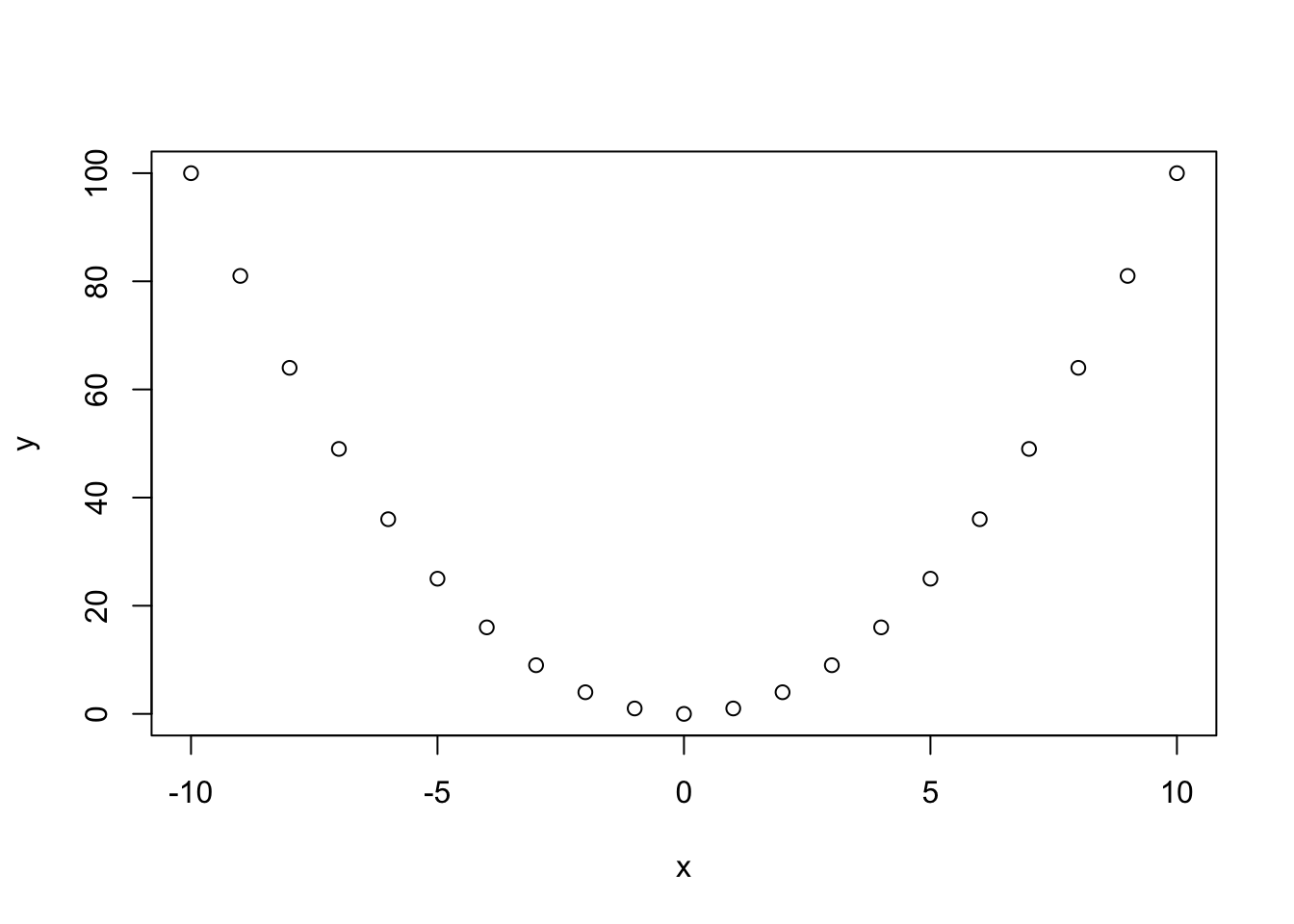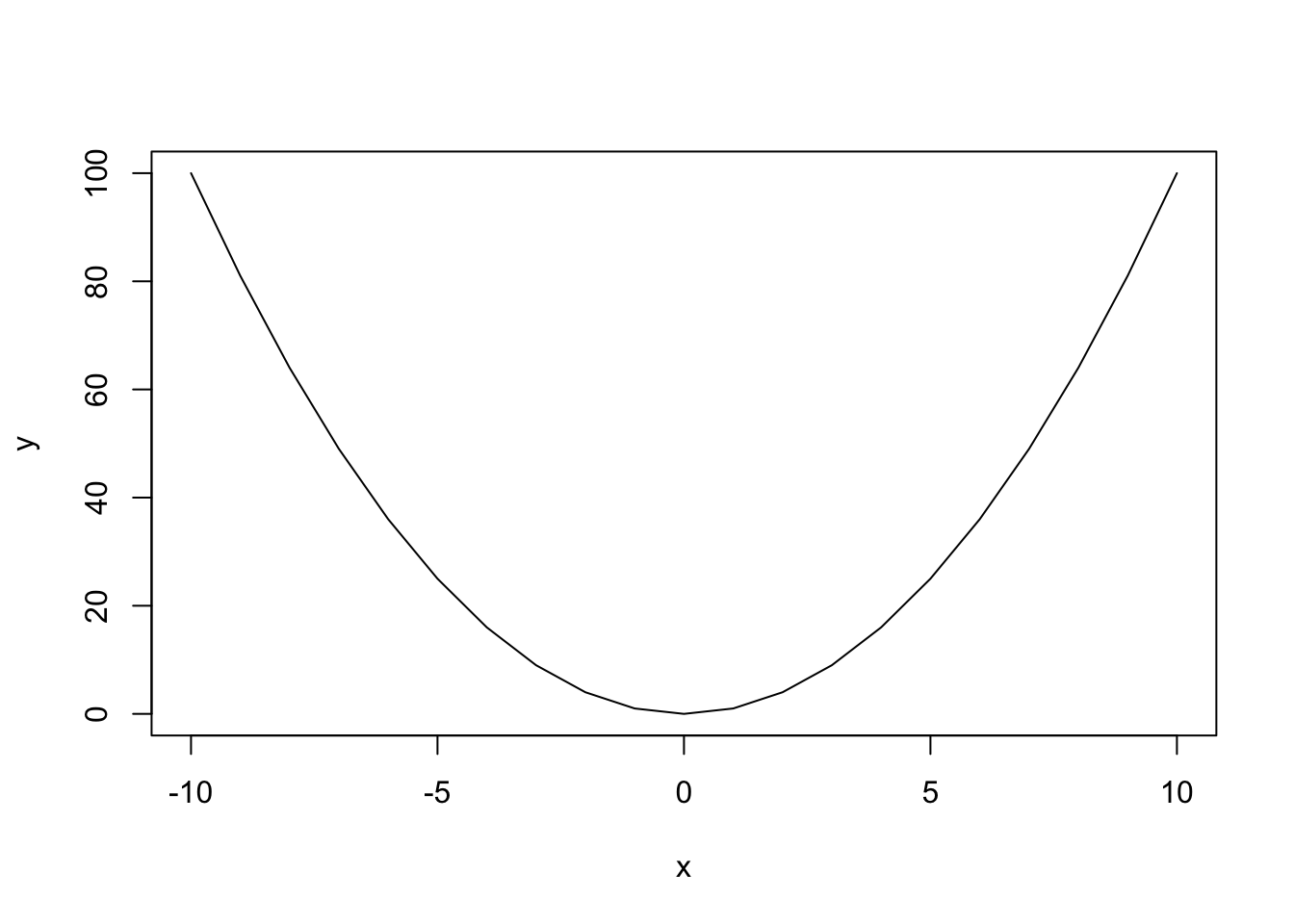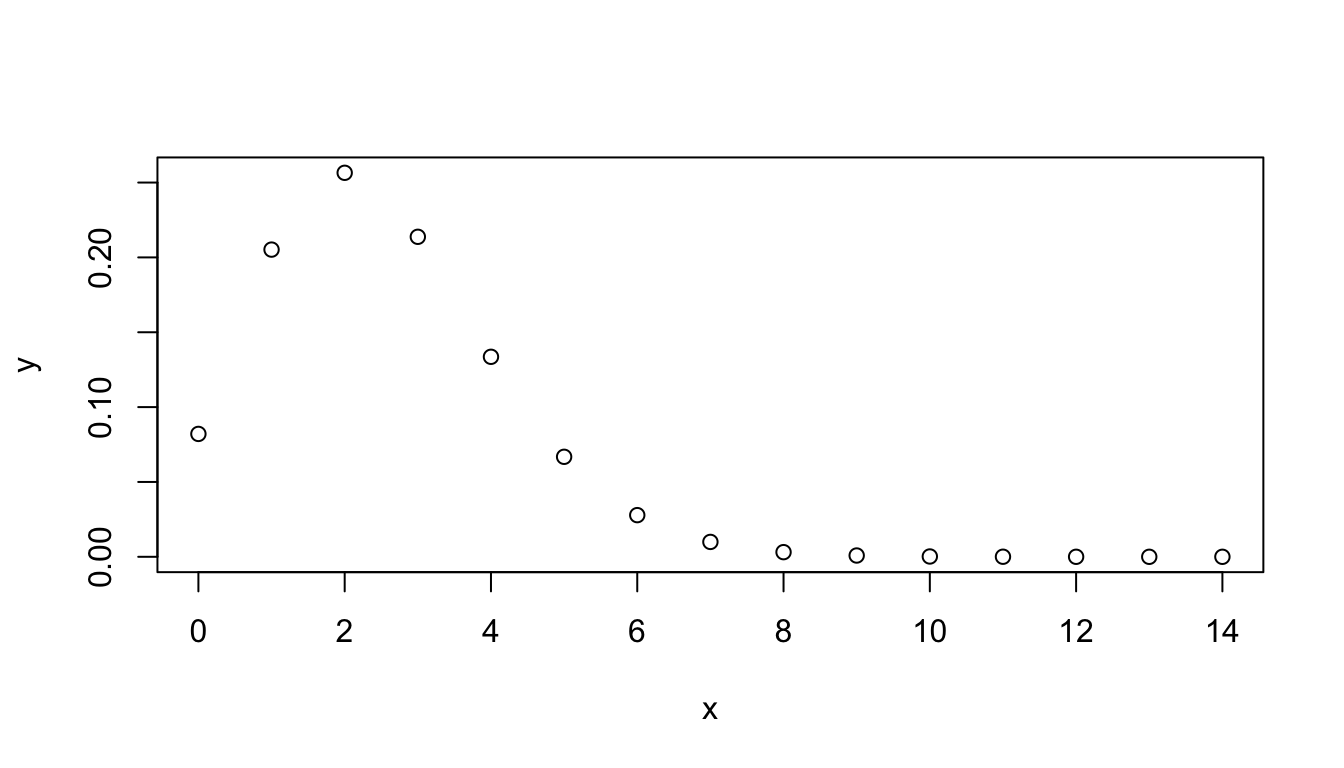2017-09-12

# Scope

In this session we will explore basic manipulations of variables.

• Assigning a value to a variable
• Basic operations on numbers

# R is a calculator

Convention:

• Dark boxes: commands to type in RStudio Console (bottom-left panel).
• White boxes: the result you should obtain.

2 + 5
 7

# Assign a value to a variable

In R <- means “create a variable and assign its value.”

Example:

• create a variable named $$a$$,
• assign the value $$2$$ to this variable,
• print the result.
a <- 2
print(a)
 2

# Computing with variables

Example:

• create a variable named $$b$$ having value $$5$$,
• compute $$a + b$$ and store the result in a variable named $$c$$,
• print the result.
b <- 5
c <- a + b
print(c)
 7

# Variables need to be updated

Example:

• change the value of $$a$$ to $$3$$,
• print the value of $$c$$
• Is this the correct result for $$c = a + b$$? Why?
a <- 3 ## Change the value of a
print(c) ## Print the value of c
 7
## Check whether c equals a + b
c == a + b
 FALSE

Note: == is used to test whether two variables have the same content.

# Updating variable contents

Example:

• change the value of $$a$$ to $$27$$,
• recompute and print the value of $$c$$
a <- 27 ## Change the value of a
c <- a + b
print(c) ## Print the value of c
 32
## Check whether c  equals a + b
c == a + b
 TRUE

# Vectors of values

The simplest data structure in R is a vector. In the previous example, the variable a was actually a vector with a single value.

Example: create a variable named three.numbers, and initialize it with a vector with values 27, 12 and 3000.

Tips: - variable names can comprize several parts, separated by dots. - the function c() combines several values into a vector

three.numbers <- c(27,12,3000)
print(three.numbers)
   27   12 3000

# Series

The simple way to create a series of numbers. The column operator permits to generate all integer values between two limits.

x <- 0:14
print(x)
   0  1  2  3  4  5  6  7  8  9 10 11 12 13 14

# Computing with vectors

R handles vectors in a very convenient way. An operation on a vector applies to all its elements.

x <- 1:10 # Define a series from 1 to 10
print(x)
   1  2  3  4  5  6  7  8  9 10
y <- x^2 # Compute the square of each number
print(y)
    1   4   9  16  25  36  49  64  81 100

# Scatter plot

x <- -10:10
y <- x^2
plot(x,y)# Line plot

x <- -10:10
y <- x^2
plot(x,y, type="l")# Variables can also contain strings

# The # symbol allows to insert comments in R code

# Define  a vector named "whoami", and
# containing two names
whoami <- c("Denis", "Siméon")
print(whoami) # Comment at the end of a line
 "Denis"  "Siméon"

# String concatenation

# Define  a vector named "names", and
# containing two names
whoami <- c("Denis", "Siméon")

# Paste the values of a vector of string
print(paste(sep=" ", whoami, whoami))
 "Denis Siméon"

# Carl’s preferred distribution

The function dpois() computes the Poisson density, i.e. the probability to observe exactly $$x$$ successes in a series of independent trials with equal probability.

The Poisson distribution is defined by a single parameter: the expected number of successes $$\lambda$$ (read “lambda”).

$P(X=x) = \frac{e^{-\lambda}\lambda^x}{x!}$

x <- 0:14   # Define the X values from 0 to 14
y <- dpois(x, lambda = 2.5) # Poisson density
print(y) # Check the result

# Plotting the Poisson distribution

x <- 0:14   # Define the X values from 0 to 14
y <- dpois(x, lambda = 2.5) # Poisson density
plot(x,y) # Check the resultThis first plot is not very nice. Let us get some help to improve it.

# Getting help for R functions

Need help? Type help().

help(plot)

A question? Type ?

?plot

Result: R displays the help message for the function dpois().

# Exercise: improve Poisson density plot

1. Do not (yet) look the next slide.
2. Read the help page for the dpois()function.
3. draw a plot that provides a didactic illustration of the Poisson density.

# Improve the plot: type = histogram

x <- 0:14
lambda <- 2.54
y <- dpois(x, lambda)
plot(x,y, type="h")